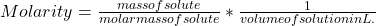## A chemistry student must write down in her lab notebook the concentration of a solution of sodium thiosulfate. The concentration of a soluti

Question

A chemistry student must write down in her lab notebook the concentration of a solution of sodium thiosulfate. The concentration of a solution equals the mass of what’s dissolved divided by the total volume of the solution.

in progress 0
4 months 2021-07-14T04:15:38+00:00 1 Answers 7 views 0

A chemistry student must write down in her lab notebook the concentration of a solution of sodium thiosulfate. The concentration of a solution equals the mass of what’s dissolved divided by the total volume of the solution.

Explanation:

The concentration of a solution can be measured in terms of molarity.

The molarity of a solution can be defined as the number of moles of solute present in the total volume of the solution.

The number of moles of solute is the ratio of mass of solute to molar mass of solute.

Hence,